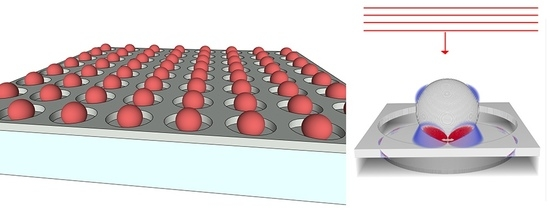Next Article in Journal
Smart Metamaterial Based on the Simplex Tensegrity Pattern
Next Article in Special Issue
Plasmonic Nanowires for Wide Wavelength Range Molecular Sensing
Open AccessArticle

# The Design and Optimization of Plasmonic Crystals for Surface Enhanced Raman Spectroscopy Using the Finite Difference Time Domain Method

byAlec Bigness andJason Montgomery *Department of Chemistry, Biochemistry, and Physics, Florida Southern College, Lakeland, FL 33801, USA
*
Author to whom correspondence should be addressed.
Materials 2018, 11(5), 672; https://doi.org/10.3390/ma11050672
Received: 30 March 2018 / Revised: 19 April 2018 / Accepted: 24 April 2018 / Published: 26 April 2018
We present computational studies of quasi three-dimensional nanowell (NW) and nanopost (NP) plasmonic crystals for applications in surface enhanced Raman spectroscopy (SERS). The NW and NP plasmonic crystals are metal coated arrays of cylindrical voids or posts, respectively, in a dielectric substrate characterized by a well/post diameter (D), relief depth ($R D$), periodicity (P), and metal thickness ($M T$). Each plasmonic crystal is modeled using the three-dimensional finite-difference time-domain (FDTD) method with periodic boundary conditions in the x- and y-directions applied to a computational unit cell to simulate the effect of a periodic array. Relative SERS responses are calculated from time-averaged electric field intensity enhancements at $λ exc$ and $λ scat$ or at $λ mid$ via $G SERS 4 = g 2 ( λ exc ) × g 2 ( λ scat )$ or $G mid 4 = g 4 ( λ mid )$ , respectively, where $g 2 = | E | 2 / | E 0 | 2$ . Comparisons of $G SERS 4$ and $G mid 4$ are made to previously reported experimental SERS measurements for NW and NP geometries. Optimized NW and NP configurations based on variations of D, P, $R D$, and $M T$ using $G SERS 4$ are presented, with 6× and 2× predicted increases in SERS, respectively. A novel plasmonic crystal based on square NP geometries are considered with an additional 3× increase over the optimized cylindrical NP geometry. NW geometries with imbedded spherical gold nanoparticles are considered, with 10× to 10$3 ×$ increases in SERS responses over the NW geometry alone. The results promote the use of FDTD as a viable in silico route to the design and optimization of SERS active devices. View Full-Text
Show FiguresGraphical abstract

MDPI and ACS Style

Bigness, A.; Montgomery, J. The Design and Optimization of Plasmonic Crystals for Surface Enhanced Raman Spectroscopy Using the Finite Difference Time Domain Method. Materials 2018, 11, 672.